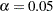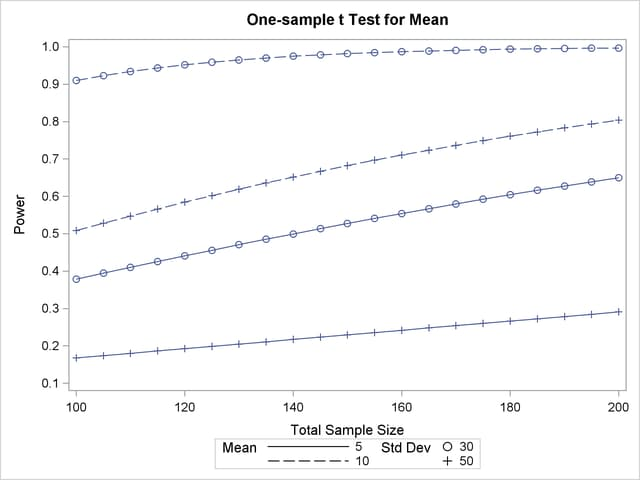Computing Power for a One-Sample t Test

Suppose you want to improve the accuracy of a machine used to print logos on sports jerseys. The logo placement has an inherently high variability, but the horizontal alignment of the machine can be adjusted. The operator agrees to pay for a costly adjustment if you can establish a nonzero mean horizontal displacement in either direction with high confidence. You have 150 jerseys at your disposal to measure, and you want to determine your chances of a significant result (power) by using a one-sampletest with a two-sided.

You decide that 8 mm is the smallest displacement worth addressing. Hence, you will assume a true mean of 8 in the power computation. Experience indicates that the standard deviation is about 40.

Use the ONESAMPLEMEANS statement in the POWER procedure to compute the power. Indicate power as the result parameter by specifying the POWER= option with a missing value (.). Specify your conjectures for the mean and standard deviation by using the MEAN= and STDDEV= options and for the sample size by using the NTOTAL= option. The statements required to perform this analysis are as follows:

```proc power;
onesamplemeans
mean   = 8
ntotal = 150
stddev = 40
power  = .;
run;
```

Default values for the TEST=, DIST=, ALPHA=, NULLMEAN=, and SIDES= options specify a two-sidedtest for a mean of 0, assuming a normal distribution with a significance level of= 0.05.

Figure 70.1 shows the output.

Figure 70.1 Sample Size Analysis for One-Sample t Test
The POWER Procedure
One-sample t Test for Mean

Fixed Scenario Elements
Distribution Normal
Method Exact
Mean 8
Standard Deviation 40
Total Sample Size 150
Number of Sides 2
Null Mean 0
Alpha 0.05

Computed Power
Power
0.682

The power is about 0.68. In other words, there is about a 2/3 chance that thetest will produce a significant result demonstrating the machine’s average off-center displacement. This probability depends on the assumptions for the mean and standard deviation.

Now, suppose you want to account for some of your uncertainty in conjecturing the true mean and standard deviation by evaluating the power for four scenarios, using reasonable low and high values, 5 and 10 for the mean, and 30 and 50 for the standard deviation. Also, you might be able to measure more than 150 jerseys, and you would like to know under what circumstances you could get by with fewer. You want to plot power for sample sizes between 100 and 200 to visualize how sensitive the power is to changes in sample size for these four scenarios of means and standard deviations. The following statements perform this analysis:

```ods listing style=htmlbluecml;
ods graphics on;

proc power;
onesamplemeans
mean   = 5 10
ntotal = 150
stddev =  30 50
power  = .;
plot x=n min=100 max=200;
run;

ods graphics off;
```

The new mean and standard deviation values are specified by using the MEAN= and STDDEV= options in the ONESAMPLEMEANS statement. The PLOT statement with X=N produces a plot with sample size on the X axis. (The result parameter, in this case the power, is always plotted on the other axis.) The MIN= and MAX= options in the PLOT statement determine the sample size range. The ODS GRAPHICS ON statement enables ODS Graphics. The ODS LISTING STYLE=HTMLBLUECML statement specifies the HTMLBLUECML style, which is suitable for use with PROC POWER because it allows both marker symbols and line styles to vary. See the section ODS Styles Suitable for Use with PROC POWER for more information.

Figure 70.2 shows the output, and Figure 70.3 shows the plot.

Figure 70.2 Sample Size Analysis for One-Sample t Test with Input Ranges
The POWER Procedure
One-sample t Test for Mean

Fixed Scenario Elements
Distribution Normal
Method Exact
Total Sample Size 150
Number of Sides 2
Null Mean 0
Alpha 0.05

Computed Power
Index Mean Std Dev Power
1 5 30 0.527
2 5 50 0.229
3 10 30 0.982
4 10 50 0.682

Figure 70.3 Plot of Power versus Sample Size for One-Sample t Test with Input RangesThe power ranges from about 0.23 to 0.98 for a sample size of 150 depending on the mean and standard deviation. In Figure 70.3, the line style identifies the mean, and the plotting symbol identifies the standard deviation. The locations of plotting symbols indicate computed powers; the curves are linear interpolations of these points. The plot suggests sufficient power for a mean of 10 and standard deviation of 30 (for any of the sample sizes) but insufficient power for the other three scenarios.